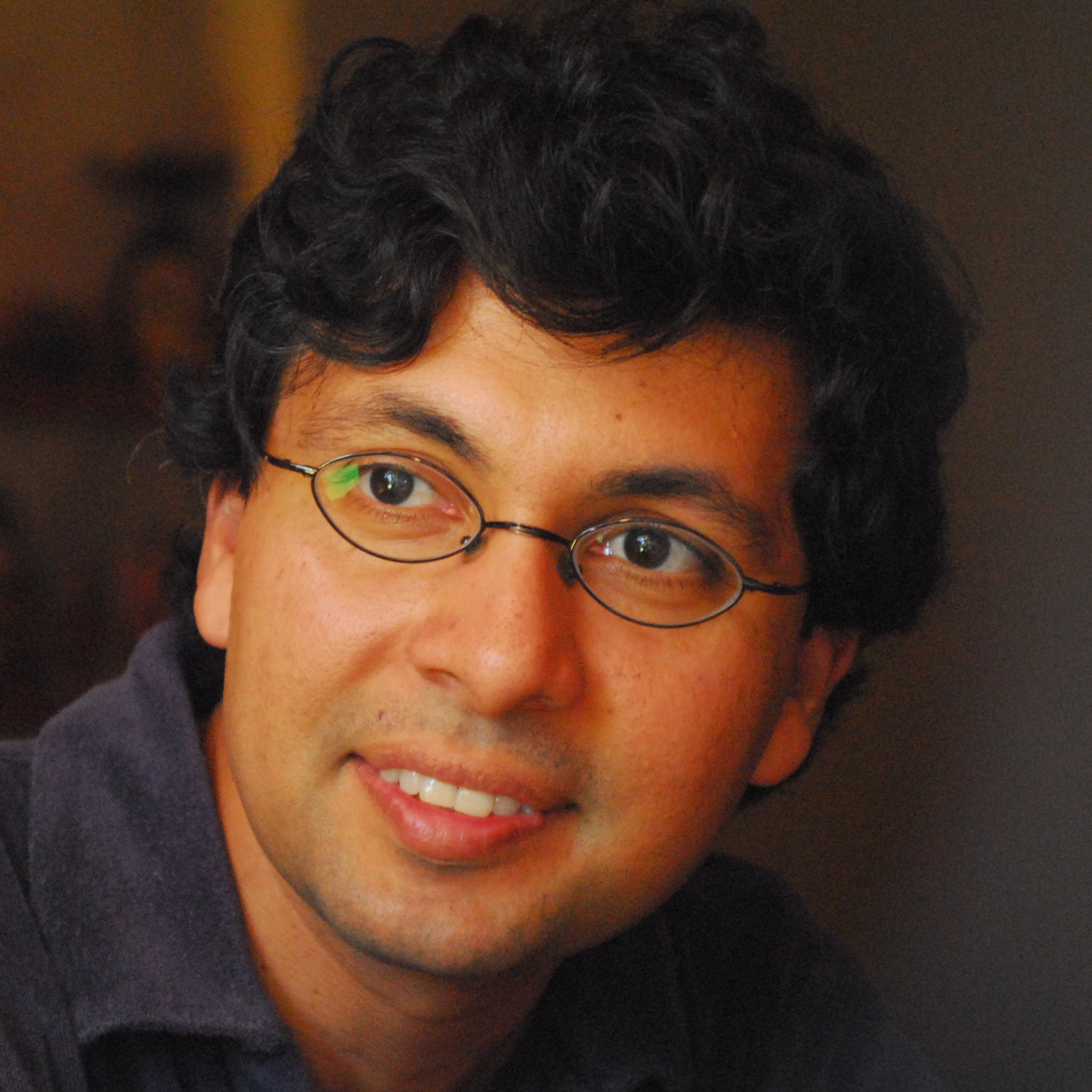## Rahul Pandharipande Department of Mathematics ETH Zürich

My papers can be found on the arXiv. Most recent papers are linked below.
Beginning students might benefit from these more introductory writings.

Two papers on localization and Hodge integrals:

I.     Localization of virtual classes (with T. Graber)
II.   Hodge integrals and Gromov-Witten theory (with C. Faber)

Papers on tautological classes:

I.     Constructions of non-tautological classes (with T. Graber)
II.   Relative maps and tautological classes (with C. Faber)
III.   New topological recursion relations (with X. Liu)
IV.   Tautological and non-tautological cohomology (with C. Faber)
V.     CohFTs with non-tautological classes (with D. Zvonkine and Appendix by D. Petersen)
VI.   Zero cycles on the moduli space of curves (with J. Schmitt)
VII.   λg, 0-sections, and the log Chow ring of the moduli of curves (with S. Molcho and J. Schmitt)

Gromov-Witten theory, Hurwitz numbers, and matrix models (with A. Okounkov)
A Fock space approach to Severi degrees (with Y. Cooper)

A trilogy (with A. Okounkov) on the Gromov-Witten theory of target curves: I, II, III

The papers fully determine the Gromov-Witten theory of target curves.
A calculator has been written by J. Bryan.

Gromov-Witten theory of complete intersections (with H. Argüz, P. Bousseau, and D. Zvonkine)

Conjectures equating the Gromov-Witten theory of 3-folds with the holomorphic Chern-Simons theory of
Donaldson-Thomas (with D. Maulik, N. Nekrasov, and A. Okounkov): I, II

Results related to the local theories of curves:

I.     Local Gromov-Witten theory (with J. Bryan)
II.   Quantum cohomology of the Hilbert scheme of points of the plane (with A. Okounkov)
III.   Local Donaldson-Thomas theory (with A. Okounkov)
IV.   QDE of the Hilbert scheme of points of the plane (with A. Okounkov)
V.     Higher genus Gromov-Witten theory of the Hilbert scheme of points of the plane (with H.-H. Tseng)
VI.   Descendents and Fourier-Mukai (with H.-H. Tseng)

Gromov-Witten/Donaldson-Thomas correspondence for toric 3-folds (with D. Maulik, A. Oblomkov, and A. Okounkov)
GW/PT descendent correspondence via vertex operators (with A. Oblomkov and A. Okounkov)
Virasoro constraints for stable pairs on toric 3-folds (with M. Moreira, A. Oblomkov, and A. Okounkov)

A topological view of Gromov-Witten theory (with D. Maulik)
New calculations in Gromov-Witten theory (with D. Maulik)

Gromov-Witten theory and Noether-Lefschetz theory (with D. Maulik)
Noether-Lefschetz theory and the Yau-Zaslow conjecture (with A. Klemm, D. Maulik, and E. Scheidegger)
Curves on K3 surfaces and modular forms (with D. Maulik and R. Thomas)
Katz-Klemm-Vafa conjecture for K3 surfaces (with R. Thomas)
Motivic stable pairs invariants for K3 surfaces (with S. Katz and A. Klemm)
Curve counting on K3xE, Igusa cusp forms, and descendent integration (with G. Oberdieck)
Curve counting on abelian varieties (with J. Bryan, G. Oberdieck, and Q. Yin)

Curve counting via stable pairs in the derived category (with R. Thomas)
The 3-fold vertex via stable pairs (with R. Thomas)
Stable pairs and BPS invariants (with R. Thomas)
13/2 ways of counting curves (with R. Thomas)

Descendents for stable pairs on local curves: rationality (with A. Pixton)
Descendents for stable pairs on local curves: stationary theory (with A. Pixton)
Descendents for stable pairs on toric 3-folds (with A. Pixton)
Gromov-Witten/Pairs descendent correspondence for toric varieties (with A. Pixton)
Gromov-Witten/Pairs correspondence for the quintic Calabi-Yau (with A. Pixton)
Descendents for stable pairs on 3-folds

Counting disks on the quintic 3-fold (with J. Solomon and J. Walcher)
Intersection theory on the moduli of disks, KdV, and Virasoro (with J. Solomon and R. Tessler)

Enumerative geometry of Calabi-Yau 4-folds (with A. Klemm)
Enumerative geometry of Calabi-Yau 5-folds, Appendix (with A. Zinger)

Algebraic cobordism revisited (with M. Levine)
Algebraic cobordism for bundles on varieties (with Y.-P. Lee)

Abelian Hurwitz-Hodge integrals (with P. Johnson and H.-H. Tseng)

Tropical vertex group (with M. Gross and B. Siebert)
Quivers, curves, and the tropical vertex (with M. Gross)

Moduli of stable quotients (with A. Marian and D. Oprea)
Stable quotients and the holomorphic anomaly equation (with H. Lho)
Holomorphic anomaly equations for the formal quintic (with H. Lho)
Crepant resolution and the holomorphic anomaly equation for [C3/Z3] (with H. Lho)

Papers on tautological rings of the moduli of curves of compact type: I, II, revised
Related results on tautological rings of the moduli of nonsingular curves (with A. Pixton)
Relations on the moduli space of stable curves via 3-spin structures (with A. Pixton and D. Zvonkine)
Hypergeometric series A and B (with A. Buryak and F. Janda)
A calculus for the moduli space of curves
Cohomological field theory calculations

Moduli of twisted canonical divisors (with G. Farkas)
Appendix on the weighted fundamental class (with F. Janda, A. Pixton, and D. Zvonkine)
Double ramification cycles (with F. Janda, A. Pixton, and D. Zvonkine)
Double ramification cycles for target varieties (with F. Janda, A. Pixton, and D. Zvonkine)
Tautological relations via r-spin structures (with A. Pixton and D. Zvonkine)
Appendix on the class of the locus of holomorphic differentials (with F. Janda, A. Pixton, and D. Zvonkine)
Universal twisted double ramification cycles (with Y. Bae, D. Holmes, J. Schmitt, and R. Schwarz)
Logarithmic double ramification cycles (with D. Holmes, S. Molcho, A. Pixton, and J. Schmitt)

Segre classes and Hilbert schemes of points (with A. Marian and D. Oprea)
On Lehn's conjecture in rank 1 and higher rank (with A. Marian and D. Oprea)
Relations in the tautological ring of the moduli of K3 surfaces (with Q. Yin)

Virtual Euler characteristics of Quot schemes of surfaces (with D. Oprea)
Rationality of descendent series for Quot schemes of surfaces (with D. Johnson and D. Oprea)
The virtual K-theory of Quot schemes of surfaces (with N. Arbesfeld, D. Johnson, W. Lim, and D. Oprea)

Slopes of the Verlinde bundles (with A. Marian and D. Oprea)
Chern characters of the Verlinde bundles (with A. Marian, D. Oprea, A. Pixton, and D. Zvonkine)

Tevelev degrees and Hurwitz moduli (with A. Cela and J. Schmitt)
Enumerativity of virtual Tevelev degrees (with C. Lian)
Tevelev degrees and Gromov-Witten theory (with A. Buch)

Return